JEE  >  Test: MCQs (One or More Correct Option): Equilibrium | JEE Advanced

# Test: MCQs (One or More Correct Option): Equilibrium | JEE Advanced - JEE

Test Description

## 12 Questions MCQ Test Chemistry 35 Year Past year Papers JEE Main & Advanced - Test: MCQs (One or More Correct Option): Equilibrium | JEE Advanced

Test: MCQs (One or More Correct Option): Equilibrium | JEE Advanced for JEE 2023 is part of Chemistry 35 Year Past year Papers JEE Main & Advanced preparation. The Test: MCQs (One or More Correct Option): Equilibrium | JEE Advanced questions and answers have been prepared according to the JEE exam syllabus.The Test: MCQs (One or More Correct Option): Equilibrium | JEE Advanced MCQs are made for JEE 2023 Exam. Find important definitions, questions, notes, meanings, examples, exercises, MCQs and online tests for Test: MCQs (One or More Correct Option): Equilibrium | JEE Advanced below.
 1 Crore+ students have signed up on EduRev. Have you?
*Multiple options can be correct
Test: MCQs (One or More Correct Option): Equilibrium | JEE Advanced - Question 1

### For the gas phase reaction :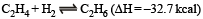carried out in a vessel, the equilibrium concentration of C2H4 can be increased by :

Detailed Solution for Test: MCQs (One or More Correct Option): Equilibrium | JEE Advanced - Question 1

The reaction is exothermic, hence increasing temperature will favour backward reaction (i.e. conc. of C2H4 increases) removing H2 and adding C2H6 favours backward reaction.

*Multiple options can be correct
Test: MCQs (One or More Correct Option): Equilibrium | JEE Advanced - Question 2

### When NaNO3 is heated in a closed vessel, oxygen is liberated and NaNO2 is left behind. At equilibrium.

Detailed Solution for Test: MCQs (One or More Correct Option): Equilibrium | JEE Advanced - Question 2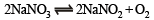According to Le-chatelier principle an increase in pressure always favours the reaction, where volume or moles decrease (i.e. reverse direction). As heat is added, i.e. reaction is endothermic and is supported in forward direction with increase in temperature.

*Multiple options can be correct
Test: MCQs (One or More Correct Option): Equilibrium | JEE Advanced - Question 3

### The equilibrium :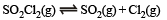is attained at 25ºC in a closed container and an inert gas, helium is introduced. Which of the following statements are correct?

Detailed Solution for Test: MCQs (One or More Correct Option): Equilibrium | JEE Advanced - Question 3

At constant volume, concentrations do not change.

*Multiple options can be correct
Test: MCQs (One or More Correct Option): Equilibrium | JEE Advanced - Question 4

For the reaction :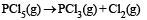The forward reaction at constant temperature is favoured by

Detailed Solution for Test: MCQs (One or More Correct Option): Equilibrium | JEE Advanced - Question 4

Introduction of an inert gas at constant pressure causes the equilibrium to shift in a direction in which number of moles increases. The forward reaction is further accelerated by increase in the quantity of substrate, i.e., PCl5 and by the increase of space, i.e., volume of container.

*Multiple options can be correct
Test: MCQs (One or More Correct Option): Equilibrium | JEE Advanced - Question 5

For the reaction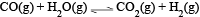at a given temperature the equilibrium amount of CO2(g) can be increased by

Detailed Solution for Test: MCQs (One or More Correct Option): Equilibrium | JEE Advanced - Question 5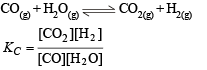A catalyst simply helps in attaining the equilibrium earlier.
Addition of inert gas has no effect on a reaction because in it Δn = 0.
This equilibrium is not based upon volume because in it  Δn = 0.
On increasing the amount of CO, KC will decrease but it is constant at constant temperature, so for maintaining the constant value of KC, the amount of CO2 increases.

*Multiple options can be correct
Test: MCQs (One or More Correct Option): Equilibrium | JEE Advanced - Question 6

Which of the following statements(s) is (are) correct?

Detailed Solution for Test: MCQs (One or More Correct Option): Equilibrium | JEE Advanced - Question 6

pH of 1 x 10-8 M is below 7 because it is an acid.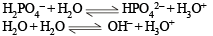K (Auto protolysis constant of water i.e. ionic product of water) increases with temperature. For half neutralisation of a weak acid by a weak base,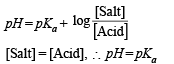*Multiple options can be correct
Test: MCQs (One or More Correct Option): Equilibrium | JEE Advanced - Question 7

A buffer solution can be prepared from a mixture of

Detailed Solution for Test: MCQs (One or More Correct Option): Equilibrium | JEE Advanced - Question 7

A buffer solution is prepared by mixing a weak acid/ base with salt of its conjugate base/acid.

*Multiple options can be correct
Test: MCQs (One or More Correct Option): Equilibrium | JEE Advanced - Question 8

Aqueous solutions of HNO3 , KOH, CH3COOH and CH3COONa of identical concentrations are provided. The pair(s) of solutions which form a buffer upon mixing is(are)

Detailed Solution for Test: MCQs (One or More Correct Option): Equilibrium | JEE Advanced - Question 8

Any solution of a weak acid and its salt with strong base acts as an acidic buffer solution.
If volume of HNO3 solution added is less as compared to that of CH3COONa solution, it results in the formation of an acidic buffer solution.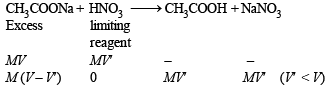*Multiple options can be correct
Test: MCQs (One or More Correct Option): Equilibrium | JEE Advanced - Question 9

The initial rate of hydrolysis of methyl acetate (1M) by a weak acid (HA, 1M) is 1/100th of that of a strong acid (HX, 1M), at 25°C. The Ka of HA is

Detailed Solution for Test: MCQs (One or More Correct Option): Equilibrium | JEE Advanced - Question 9

As ester hydrolysis is first order with respect [H+].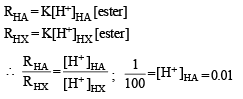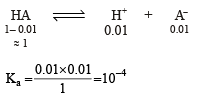*Multiple options can be correct
Test: MCQs (One or More Correct Option): Equilibrium | JEE Advanced - Question 10

The Ksp of Ag2CrO4 is 1.1 × 10–12 at 298 K. The solubility (in mol/L) of Ag2CrO4 in a 0.1 M AgNO3 solution is

Detailed Solution for Test: MCQs (One or More Correct Option): Equilibrium | JEE Advanced - Question 10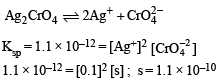*Multiple options can be correct
Test: MCQs (One or More Correct Option): Equilibrium | JEE Advanced - Question 11

The thermal dissociation equilibrium of CaCO3(s) is studied under different conditions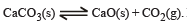For this equilibrium, the correct statement(s) is(are)

Detailed Solution for Test: MCQs (One or More Correct Option): Equilibrium | JEE Advanced - Question 11Hence enthalpy depends on temperature.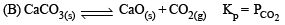(C) Keq depends only on temperature and not on Pressure.
(D) Enthalpy of reaction is independent of the catalyst.
Catalyst generaly changes activation energy.

*Multiple options can be correct
Test: MCQs (One or More Correct Option): Equilibrium | JEE Advanced - Question 12

The %yield of ammonia as a function of time in the reaction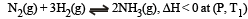is given below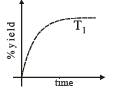If this reaction is conducted at (P, T2), with T2 > T1, the %yield of ammonia as a function of time is represented by

Detailed Solution for Test: MCQs (One or More Correct Option): Equilibrium | JEE Advanced - Question 12

Initially on increasing temperature rate of reaction will increase, so % yield will also increase with time. But at equilibrium % yield at high temperature (T2) would be less than at T1 as reaction is exothermic so the graph is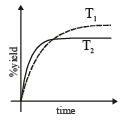## Chemistry 35 Year Past year Papers JEE Main & Advanced

142 docs|66 tests
Information about Test: MCQs (One or More Correct Option): Equilibrium | JEE Advanced Page
In this test you can find the Exam questions for Test: MCQs (One or More Correct Option): Equilibrium | JEE Advanced solved & explained in the simplest way possible. Besides giving Questions and answers for Test: MCQs (One or More Correct Option): Equilibrium | JEE Advanced, EduRev gives you an ample number of Online tests for practice

## Chemistry 35 Year Past year Papers JEE Main & Advanced

142 docs|66 tests(Scan QR code)